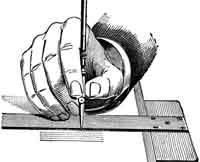#Difference Between Similar Terms and Objects

# Difference Between Dot Product and Cross ProductDot Product vs Cross Product

Dot product and cross product have several applications in physics, engineering, and mathematics. The cross product, or known as a vector product, is a binary operation on two vectors in a three-dimensional space. The cross product results in a vector that is perpendicular to both the vectors that are multiplied and normal to the plain.

In algebraic operations, the dot product takes two equal length sequences of numbers and gives a single number. It is obtained by multiplying the corresponding entries and thereafter summing the products.

If the vectors are named “a” and “b,” then the dot product is represented by “a . b.” This is equal to the magnitudes multiplied by the cosine of the angles. In vectors “a” and “b,” the cross product is represented by “a X b.” This is equal to the magnitudes multiplied by the sine of the angles and thereafter multiplied by “n,” a unit vector.

It can be noticed that the magnitude of a dot product is a maximum whereas it is zero in a cross product. Both the dot product and the cross product rely on the metric of Euclidean space. However, the cross product also relies on choice orientation.

A dot product is generally used when there is a need to project a vector onto another vector. Some of the examples of dot products are:

Calculating distance of a point to a plane.
Calculating distance of a point to a line.
Calculating projection of a point.

A cross product has many usages, such as:

Calculating distance of a point to a plane.
Calculating the specular light.

Summary:

1.The cross product or vector product is a binary operation on two vectors in a three-dimensional space.
2.In algebraic operations, the dot product takes two equal length sequences of numbers and gives a single number.
3.The cross product results in a vector that is perpendicular to both the vectors that are multiplied and normal to the plane.
4.The dot product is obtained by multiplying the corresponding entries and then summing the products.
5.The magnitude of the dot product is a maximum whereas it is zero in a cross product.
6.A dot product is generally used when there is a need to project a vector onto another vector.
7.If the vectors are named “a” and “b,” then the dot product is represented by “a . b.” In vectors “a” and “b,” the cross product is represented by “a X b.”

### Search DifferenceBetween.net :

Custom Search

Help us improve. Rate this post!(4 votes, average: 4.00 out of 5)Loading...Email This Post : If you like this article or our site. Please spread the word. Share it with your friends/family.

Please note: comment moderation is enabled and may delay your comment. There is no need to resubmit your comment.

Articles on DifferenceBetween.net are general information, and are not intended to substitute for professional advice. The information is "AS IS", "WITH ALL FAULTS". User assumes all risk of use, damage, or injury. You agree that we have no liability for any damages.